# Easy projection mapping for p5js

`p5.projection.js` is a library that hopes makes it easy to map a p5js sketch onto a projected surface to correct for 3D shapes, projector mis-alignment or even moving surfaces. It requires four `uv` coordinates and four `xy` coordinates to create the sixteen values for `applyMatrix()` that will make the projected image line up with the real world.

For many applications it is enough to click on the four corners of the projected image and map those to the canvas coordinates (0,0), (1920,0), (1920,1080) and (0,1080), then call into the `update()` function to compute the forward and inverse matrices.

Inspired by OpenCV `perspectiveTransform()`

## Getting started

Include `math.js` and `p5.projection.js` in your code and create `ProjectionMatrix` object. In your `setup()` function create a `WEBGL` canvas and in your `draw()` function, call `mat.apply()` to skew the drawing to the projected frame. You can add a `mouseClicked()` to update the `mat.outPts` array with the correct corners.

``````<html>
<script src="https://cdnjs.cloudflare.com/ajax/libs/p5.js/1.3.1/p5.js"></script>
<script src="https://cdnjs.cloudflare.com/ajax/libs/mathjs/9.5.1/math.js"></script>
<script src="https://cdn.jsdelivr.net/gh/osresearch/p5.projection@0.2.1/library/p5.projection.js"></script>
<body>
<script>
let mat = new ProjectionMatrix();

function setup()
{
createCanvas(windowWidth-10, windowHeight-10, WEBGL);
mat.edit = true;
}

function draw()
{
background(0);

mat.apply();

fill(80);
stroke(150);
strokeWeight(2);
rect(400, 200, 200, 200);
rect(600, 200, 200, 200);
rect(800, 200, 200, 200);
rect(800, 400, 200, 200);
rect(600, 600, 200, 200);
}
</script>
</body>
</html>
``````

## Examples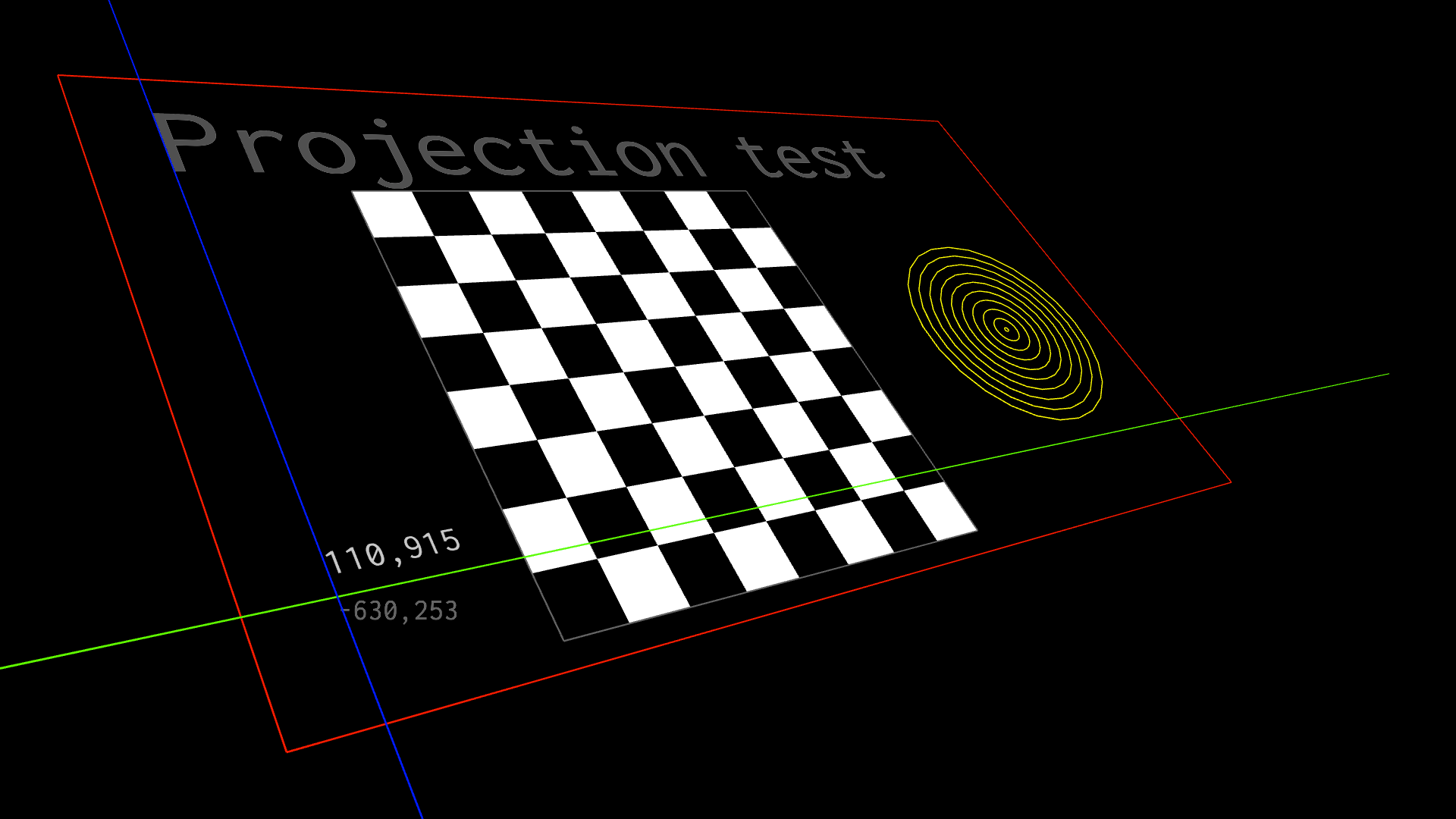Chessboard example shows how to translate screen coordinates into canvas coordinates and back, as well as demonstrates line drawing and fonts in the projection mapping mode.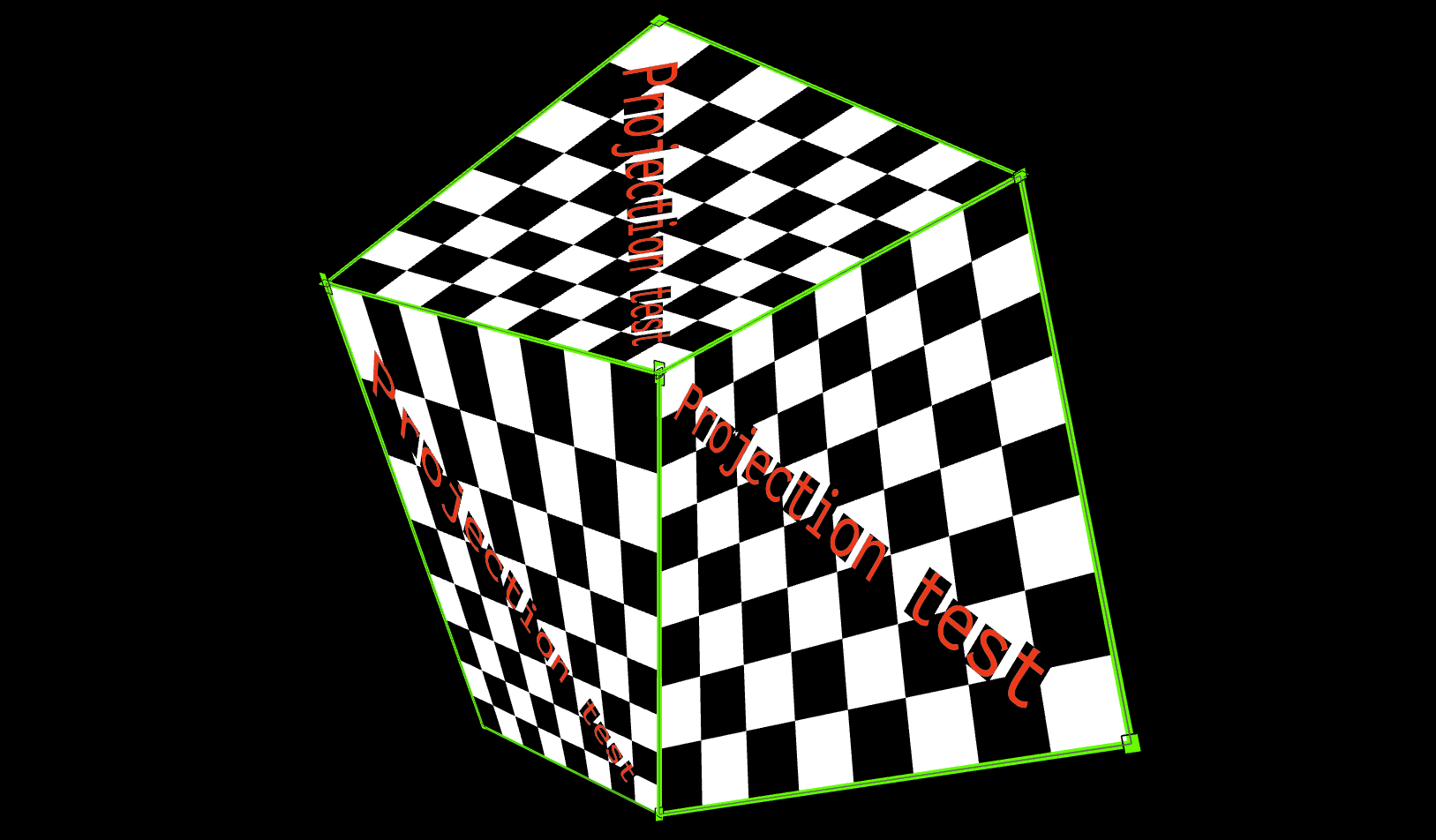Multiple projected surfaces on a cube shows how multiple `ProjectionMatrix` objects can be created to allow mapping to multiple real world surfaces, such as onto the three visible faces of a cube.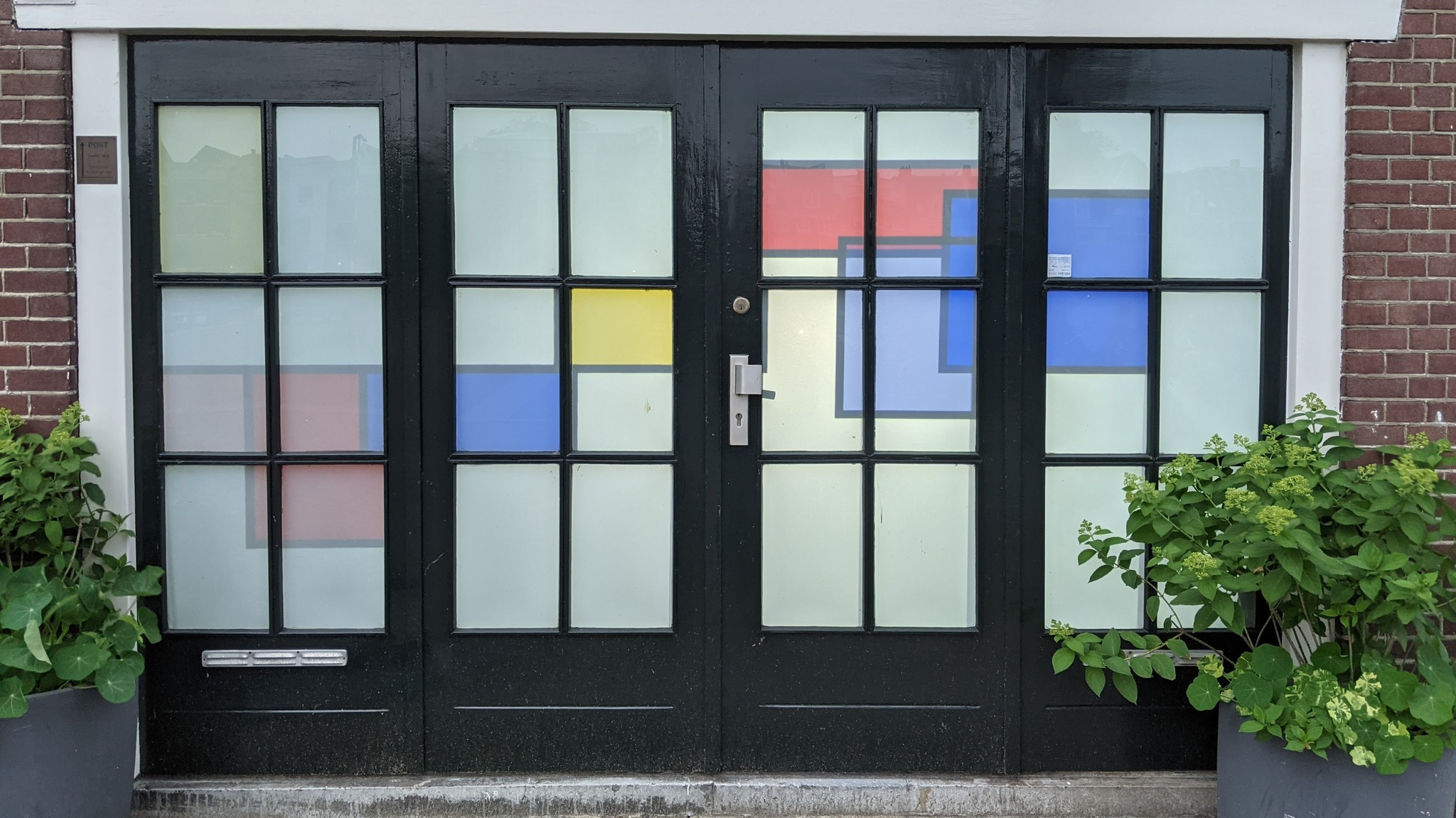Mondrian art rear-projected on glass doors. This was the art installation that started this project.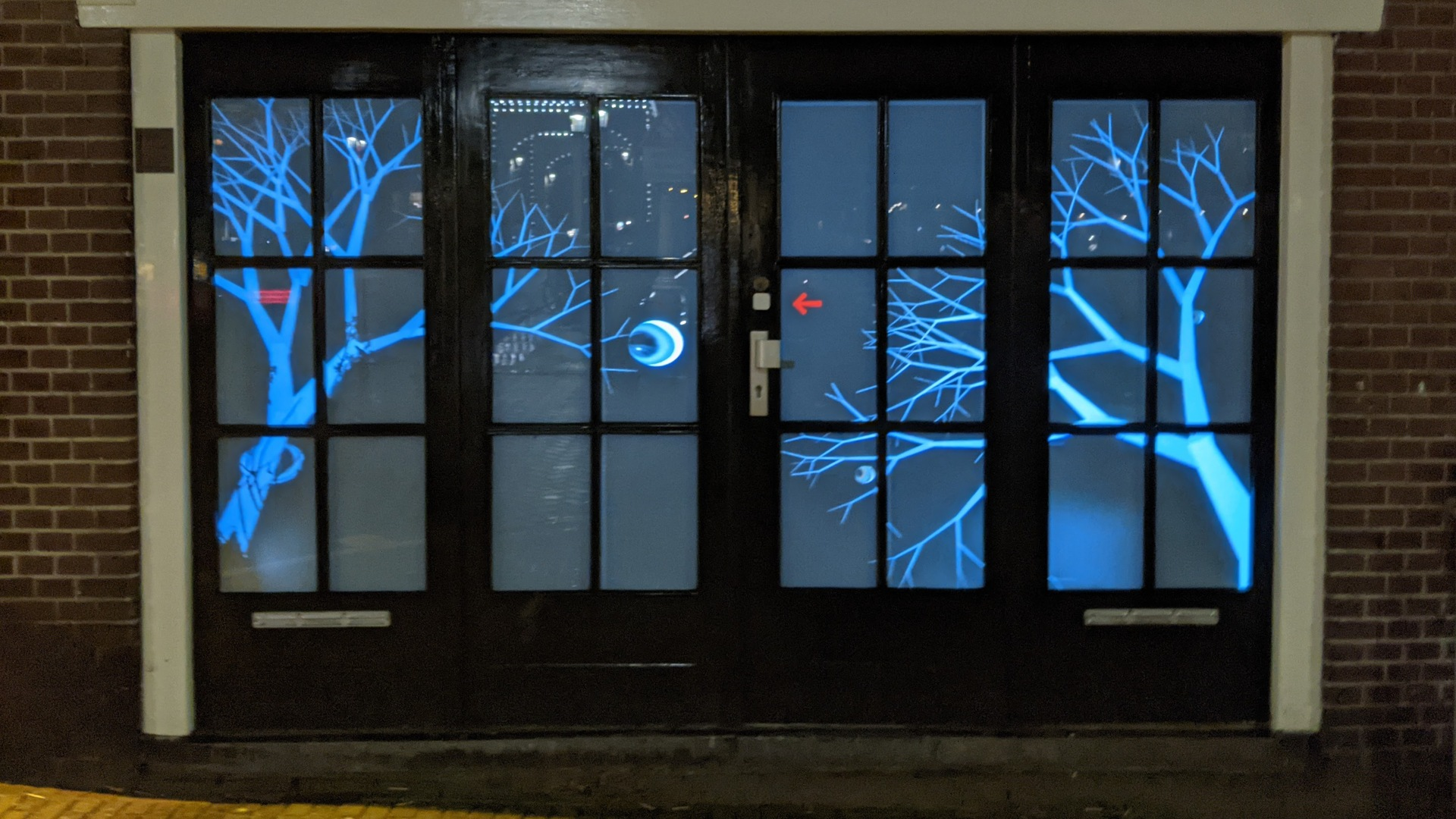Halloween trees and eyeballs, on the same glass doors.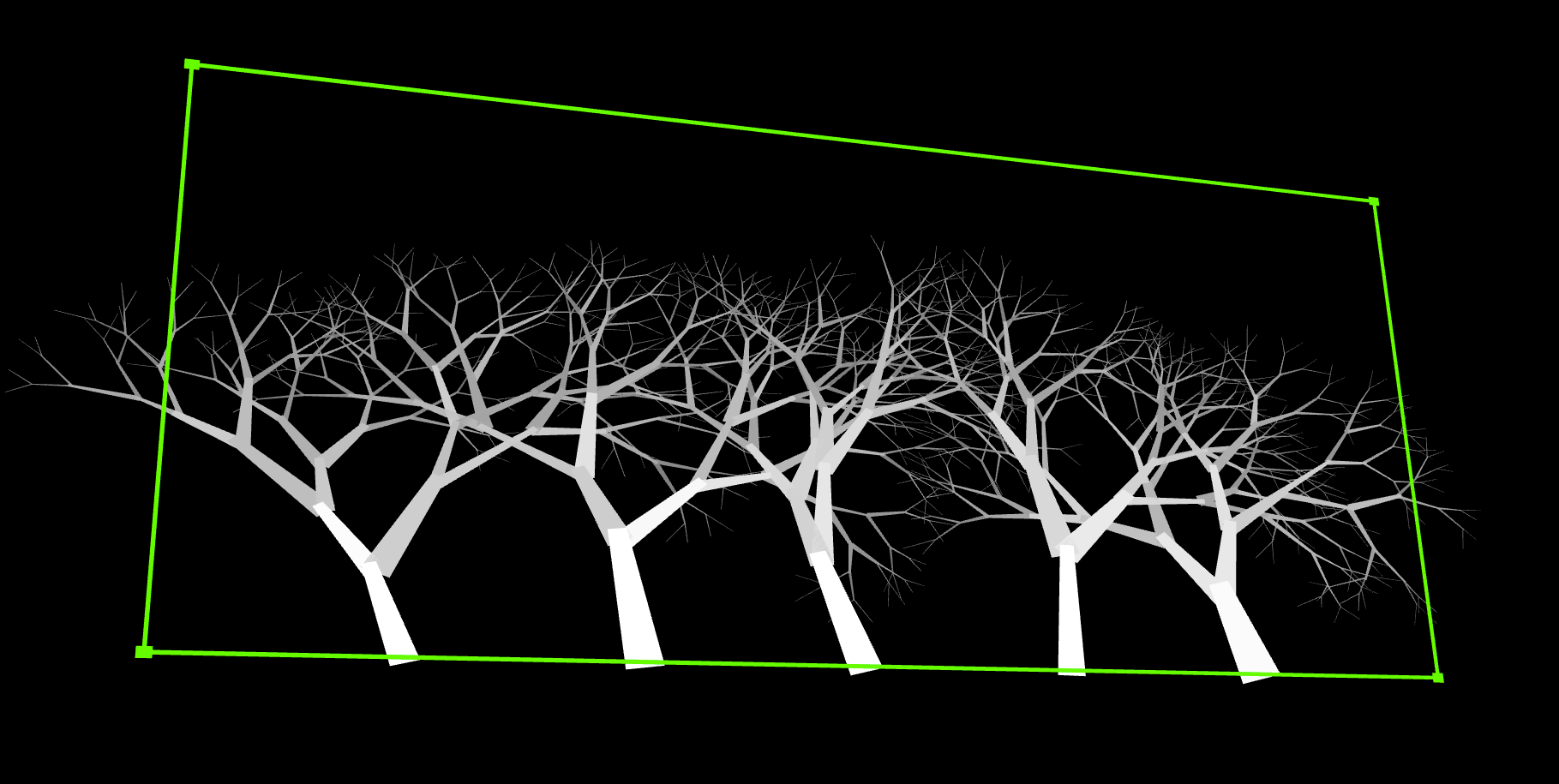Just the fractal trees, which slowly wave in a creepy way, blown by the GPU fan that spins up to draw all those line segments.

## The math parts

You don’t need to understand this part to use the library, although you might find it interesting to see how it works under the covers.The XY drawing coordinates are translated into UV screen coordinates using a normal perspective transform matrix C that maps from `(x,y)` to `(u,v,z)` space through `C xy = uvz`:

``````/ c00 c01 c02 \   / x \    / u \
| c10 c11 c12 | * | y |  = | v |
\ c20 c21 1   /   \ 1 /    \ z /
``````

Multiplying the matrix and applying the perspective transform to make `u` and `v` shrink towards the center as they get further away from the “camera”:

`````` zi =  c20*xi + c21*yi + 1
ui = (c00*xi + c01*yi + c02) / zi
vi = (c10*xi + c11*yi + c12) / zi
``````

The problem is how to find the eight variable entries in the `C` matrix so that the `xy` points correctly map to output real-world coordinates in `uv` space. Since the library takes in four `xy` points and four corresponding `uv` points, this is eight knowns which should uniquely map to the eight unknowns.

To make it easier to solve, it is best to rewrite the `uv` equations and expand them out so that the variables are isolated.

`````` ui * zi = c00*xi + c01*yi + c02
vi * zi = c10*xi + c11*yi + c12
``````

Each of these can be expanded

`````` ui * (c20*xi + c21*yi + 1) = c00*xi + c01*yi + c02
c20*(ui*xi) + c21*(ui*yi) + ui = c00*xi + c01*yi + c02
ui = c00*xi + c01*yi + c02 - c20*(ui*xi) - c21*(ui*yi)
``````

and

`````` vi * (c20*xi + c21*yi + 1) = c10*xi + c11*yi + c12
c20*(vi*xi) + c21*(vi*yi) + vi = c10*xi + c11*yi + c12
vi = c10*xi + c11*yi + c12 - c20*(vi*xi) - c21*(vi*yi)
``````

These eight equations can now be written as:

`````` u0 = c00*x0 + c01*y0 + c02 - c20*(u0*x0) - c21*(u0*y0)
u1 = c00*x1 + c01*y1 + c02 - c20*(u1*x1) - c21*(u1*y1)
u2 = c00*x2 + c01*y2 + c02 - c20*(u2*x2) - c21*(u2*y2)
u3 = c00*x3 + c01*y3 + c02 - c20*(u3*x3) - c21*(u3*y3)
v0 = c10*x0 + c11*y0 + c12 - c20*(v0*x0) - c21*(v0*y0)
v1 = c10*x1 + c11*y1 + c12 - c20*(v1*x1) - c21*(v1*y1)
v2 = c10*x2 + c11*y2 + c12 - c20*(v2*x2) - c21*(v2*y2)
v3 = c10*x3 + c11*y3 + c12 - c20*(v3*x3) - c21*(v3*y3)
``````

This is the linear system:

`````` / x0 y0  1  0  0  0 -x0*u0 -y0*u0 \ /c00\ /u0\
| x1 y1  1  0  0  0 -x1*u1 -y1*u1 | |c01| |u1|
| x2 y2  1  0  0  0 -x2*u2 -y2*u2 | |c02| |u2|
| x3 y3  1  0  0  0 -x3*u3 -y3*u3 |.|c10|=|u3|
|  0  0  0 x0 y0  1 -x0*v0 -y0*v0 | |c11| |v0|
|  0  0  0 x1 y1  1 -x1*v1 -y1*v1 | |c12| |v1|
|  0  0  0 x2 y2  1 -x2*v2 -y2*v2 | |c20| |v2|
\  0  0  0 x3 y3  1 -x3*v3 -y3*v3 / \c21/ \v3/
``````

The variables `c_ij` can be computed by inverting the 8x8 matrix, or using math.js function `lusolve()`.

## The WebGL parts

The p5 `applyMatrix()` takes either 6 elements, in which case only affine transforms are supported, or 16 elements, which allows arbitrary XYZ to UVW projections.

Since the 2D values have `z=0`, the equation that the matrix should apply is:

`````` ui = c00*xi + c01*yi + 0*zi + c02
vi = c10*xi + c11*yi + 0*zi + c12
zi = c20*xi + c21*yi + 0*zi + 1
``````

This means that the columns of the arguments to `applyMatrix()` must be `c00, c01, 0, c02`, `c10, c11, 0, c12`, and `c20, c21, 0, 1`:

``````	applyMatrix(
mat, mat, 0, mat,
mat, mat, 0, mat,
0,      0,      1, 0,
mat, mat, 0, mat);
``````

The helper function applies this matrix for the caller.